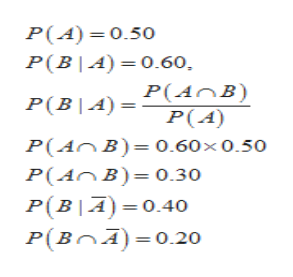# Pro basketball player Shaquille O’Neal is a poor free-throw shooter. Consider situations in which he shoots a pair of free throws. The probability that he makes the first free throw is 0.5. Given that he makes the first, suppose the probability that he makes the second is 0.60. Given that he misses the first, suppose the probability that he makes the second one is 0.40.Find the probability that he makes one of the two free throws

Question
89 views

Pro basketball player Shaquille O’Neal is a poor free-throw shooter. Consider situations in which he shoots a pair of free throws. The probability that he makes the first free throw is 0.5. Given that he makes the first, suppose the probability that he makes the second is 0.60. Given that he misses the first, suppose the probability that he makes the second one is 0.40.

Find the probability that he makes one of the two free throws

check_circle

Step 1

Let A be the event that shows the he makes the first throw success.

Similarly , B be the event that shows the he makes the second throw success.

Thus,help_outlineImage TranscriptioncloseP(A) =0.50 P(B|A)= 0.60 P(B|A)=P(AnB) P(A) P(AnB)= 0.60x0.50 P(AnB) 0.30 P(BIA) 0.40 P(BA) 0.20 fullscreen
Step 2

Hence, the required probability can be calculated as:

P( probability that the he makes one of ...

### Want to see the full answer?

See Solution

#### Want to see this answer and more?

Solutions are written by subject experts who are available 24/7. Questions are typically answered within 1 hour.*

See Solution
*Response times may vary by subject and question.
Tagged in

### Statistics